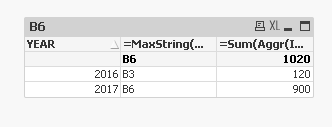# QlikView App Development

Discussion Board for collaboration related to QlikView App Development.

New Contributor II

## How to sum amount over maxstring of a dimension

Dear all,

I have several budgets (with a given amount) per year, and I need to display in a table chart the last budget amount for each year.

Example of data :

YEARBUDGET NUMBERAMOUNT
2016B1100
2016B2110
2016B3120
2017B4200
2017B5120
2017B6900

What I would like to get, based on budget number :

YEARBUDGET NUMBERAMOUNT
2016B3120
2017B6

900

I have no problem to have the correct budget number (in dimension)  :

=Aggr(MaxString([BUDGET NUMBER]), YEAR)

But I don't know how to do to get the correct amount. I tried the following expressions :

=SUM( {< [BUDGET NUMBER] ={\$(=MaxString([BUDGET NUMBER]))} >} [AMOUNT])

=Sum({\$<[BUDGET NUMBER]={'\$(=MaxString(Aggr(MaxString([BUDGET NUMBER]), [YEAR] )))'} >}[AMOUNT])**

Any help will be really appreciatedTags (2)
1 Solution

Accepted Solutions
HighlightedMVP

## Re: How to sum amount over maxstring of a dimension

Or this

Dimension

YEAR

=Aggr(If([BUDGET NUMBER] = MaxString(TOTAL <YEAR> [BUDGET NUMBER]), [BUDGET NUMBER]), YEAR, [BUDGET NUMBER])

Expression

=Sum(AMOUNT)

3 Replies
HighlightedMVP

## Re: How to sum amount over maxstring of a dimension

May be like attachedHighlightedMVP

## Re: How to sum amount over maxstring of a dimension

Or this

Dimension

YEAR

=Aggr(If([BUDGET NUMBER] = MaxString(TOTAL <YEAR> [BUDGET NUMBER]), [BUDGET NUMBER]), YEAR, [BUDGET NUMBER])

Expression

=Sum(AMOUNT)

Highlighted
New Contributor II

## Re: How to sum amount over maxstring of a dimension

Thanks a lot, it works like a charm